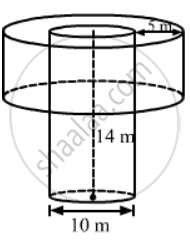Advertisement Remove all ads

# In a Village, a Well with 10 M Inside Diameter, is Dug 14 M Deep. Earth Taken Out of It is Spread All Around to a Width 5 M to Form an Embankment. Find the Height of the - Mathematics

Short Note

In a village, a well with 10 m inside diameter, is dug 14 m deep. Earth taken out of it is spread all around to a width 5 m to form an embankment. Find the height of the embankment. What value of the villagers is reflected here?

Advertisement Remove all ads

#### SolutionWe have,

Radius of well, R = 10/2 = 5 m,

Depth of the well, H = 14 m and

Width of the embarkment = 5 m,

Also, the outer radius of the embankment, r = R + 5 = 5 + 5 = 10 m

And , the inner radius of the embarkment = R = 5m

Let the height of the embankment = 5 m,

Also, the outer radius of the embankment, r = R + 5 = 5 + 5 = 10 m

And, the inner radius of the embankment = R = 5 m

Let the height of the embarkment be h.

Now,

Volume of the embankment = Volume of the earth taken out

⇒  Volume of embankment = Volume of the well

⇒ (πr2 - πR2) h = πR2H

⇒ π (r2 - R2) h = πR2H

⇒ (r2 - R2) h = R2H

⇒ (102 - 52) h = 5 × 5 × 14

⇒ (100 - 25) h = 25 × 14

⇒ 75 h = 25 × 14

⇒ "h" = (25xx14)/75

therefore  "h" = 14/3  "m"

So, the height of the embankment is 14/3 m

Value: We must lanour hard to make maximum use of the available resources.

Disclaimer: The answer provided in the textbook is incorrect. It has been corrected above.

Is there an error in this question or solution?
Advertisement Remove all ads

#### APPEARS IN

RS Aggarwal Secondary School Class 10 Maths
Chapter 19 Volume and Surface Area of Solids
Exercise 19B | Q 31 | Page 900
Advertisement Remove all ads

#### Video TutorialsVIEW ALL 

Advertisement Remove all ads
Share
Notifications

View all notifications

Forgot password?
Course